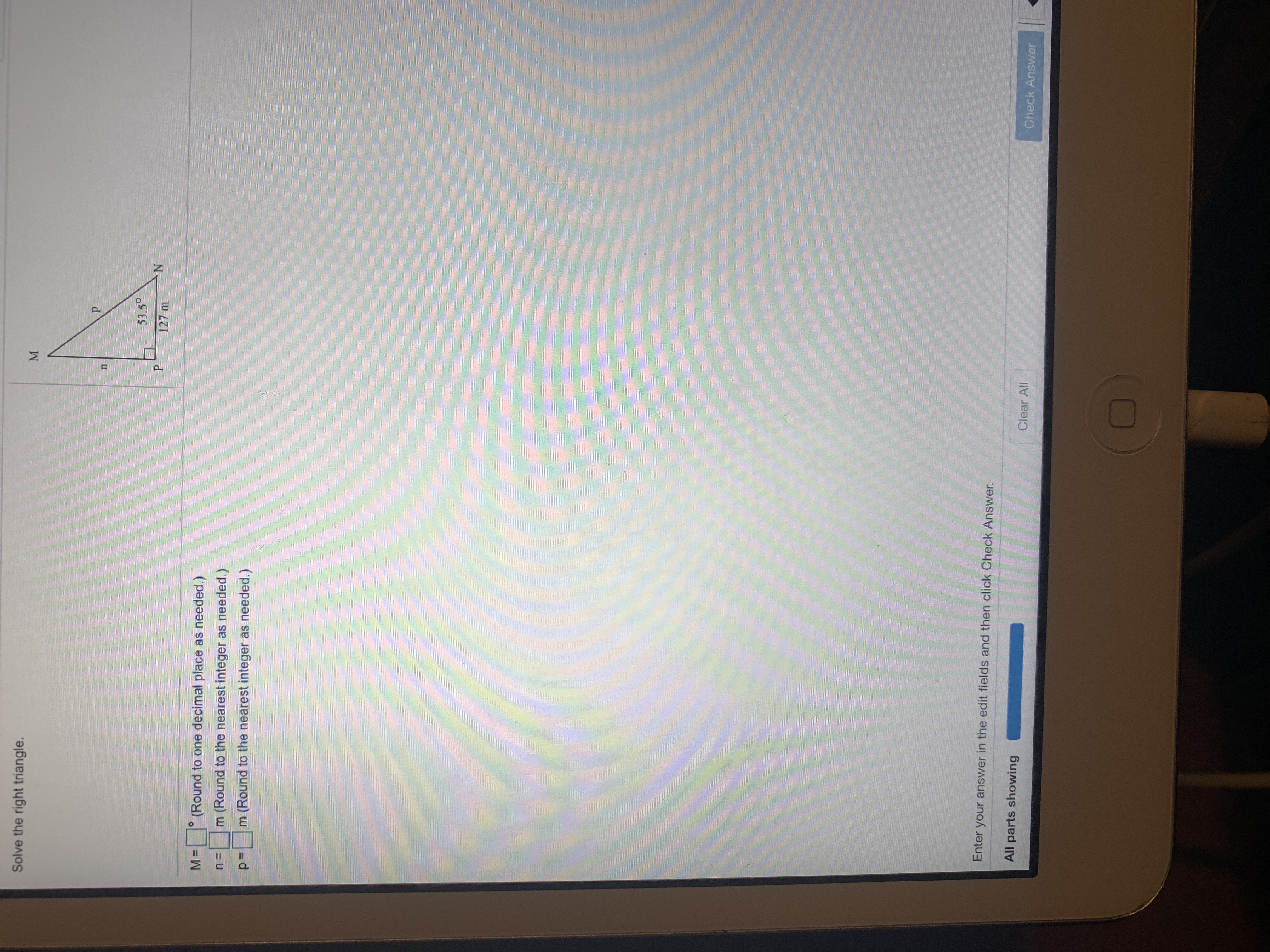# Solve the right triangle.P53.5°P127 m(Round to one decimal place as needed.)m (Round to the nearest integer as needed.)n =m (Round to the nearest integer as needed.)Enter your answer in the edit fields and then click Check Answer.All parts showingClear AllCheck AnswerIIM

Question
1 viewshelp_outlineImage TranscriptioncloseSolve the right triangle. P 53.5° P 127 m (Round to one decimal place as needed.) m (Round to the nearest integer as needed.) n = m (Round to the nearest integer as needed.) Enter your answer in the edit fields and then click Check Answer. All parts showing Clear All Check Answer II M fullscreen
check_circle

Step 1

Sum of the acute angles in right triangle = 90 degree.

One angle is N= 53.5 degree
So, M=90 degree - (53.5 degree)=36.5 degree

Step 2

Use opposite/adjacent=tan theta to find n.

Used calcul...

### Want to see the full answer?

See Solution

#### Want to see this answer and more?

Solutions are written by subject experts who are available 24/7. Questions are typically answered within 1 hour.*

See Solution
*Response times may vary by subject and question.
Tagged in

### Trigonometry